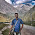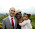## Saturday, June 2, 2012

### Visualizing car brand choices in ggplot2

I always like to read new posts at chartsnthings as they always inspire me with new ideas for data visualization. Yesterday I have read an article on choices of car brands by members of parliament in Poland in Gazeta.pl. It contains a simple table graph created using Tableau that was interesting to replicate in GNU R.
The source data (cars.txt) contains club name each member of parliament belongs to and name of the car she owns. Here is the head of the data set:

cars <- read.table("cars.txt",
head = T, sep = "\t"quote = "")
head(cars)
#   club                              brand
# 1   PO                         Citroen C8
# 2   PO Opel Astra IV Combi 1,4 Turbo 2011
# 3   PO                     Mercedes E 300
# 4   PO                   Chevrolet Blazer
# 5   PO                     Mercedes C 202
# 6   PO                         Mercedes G

In order to do the cross tabulation of clubs against car brands first I leave only brand name in the data, next I order both factors by categories count and plot the data:

library(ggplot2)
library(plyr)

# leave only car brand
cars\$brand <- factor(sapply(cars\$brand,
function(x) { strsplit(as.character(x)," ")[] }))

# order clubs and brands by counts
cars\$club <- ordered(cars\$club,
names(sort(table(cars\$club))))
cars\$brand <- ordered(cars\$brand,
names(sort(table(cars\$brand), decreasing = TRUE)))

# transform the data for plotting
scars <- ddply(cars, .(brand, club), .fun = nrow)

ggplot() +
geom_point(data = scars,
aes(x = brand, y = club, colour = log(V1)),
shape=15, size = 4) +
scale_colour_gradient(low = "#AFE9AF", high = "#0B280B") +
opts(panel.background = theme_blank(),
legend.position = "none",
axis.title.x = theme_blank(),
axis.title.y = theme_blank(),
axis.text.x = theme_text(angle = -90),
axis.text.y = theme_text(colour = "black"))

Here is the final plot:

#### 8 comments:

1.V1 is not defined in script. Can you help me?

1.V1 is a count of same (or equal) rows in the 'cars' dataset. Type in head(scars) to get a better idea.

2.You could name the variable directly for example like this:
ddply(cars, .(brand, club),
.fun = function(x) { c(count = nrow(x)) })
now its name is "count".

Using the following call:
ddply(cars, .(brand, club), "nrow")
gives the variable name nrow.

Unfortunately neither:
ddply(cars, .(brand, club), c(count="nrow"))
nor
ddply(cars, .(brand, club), list(count="nrow"))
works (although this would work for more than one output variable).

2.Excellent post, thank you! Looking forward to use something similar in my work/play

3.I like your use of shape = 15 here.

Below is code to reproduce a similar plot using the geom_tile instead of geom_point.

test2 <- ggplot(scars, aes(club, factor(brand))) + geom_tile(aes(fill = V1))
test2 <- test2 + scale_fill_gradient2(name=NULL, low="cornflowerblue", high="firebrick", midpoint = 15, trans="identity")

test2 <- test2 + labs(x = "Affiliation", y = "Brand") + opts(axis.ticks = theme_blank(), axis.text.x = theme_text(size = 10, angle = 45, hjust = 1, colour = "grey25"), axis.text.y = theme_text(size=10, colour = 'gray25'), panel.background = theme_blank())

1.Nice. This is similar to my post http://rsnippets.blogspot.com/2012/05/visualizing-tables-in-ggplot2.html.

I used shape=15 to have some white space between boxes.

4.Hi, thanks for the post. I just tried the code and I have massive white space between the clubs (using R2.15, all latest packages).

1.This is because the plot fills whole graphics area.
The simplest way to fix it is to manually resize the plotting window.